ISEE Lower Level Quantitative : Operations with fractions and whole numbers

Example Questions

Example Question #3 : Adding Mixed Numbers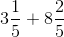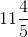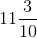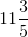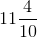Explanation:

When we add mixed numbers, we add whole numbers to whole numbers and fractions to fractions.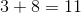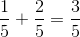Remember, when we are adding fractions we must have common denominators and we only add the numerators.

Example Question #111 : Operations With Fractions And Whole Numbers

Solve: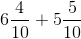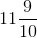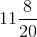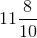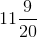Explanation:

When we add mixed numbers, we add whole numbers to whole numbers and fractions to fractions.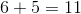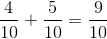Remember, when we are adding fractions we must have common denominators and we only add the numerators.

Example Question #5 : Adding Mixed Numbers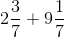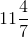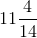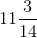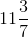Explanation:

When we add mixed numbers, we add whole numbers to whole numbers and fractions to fractions.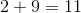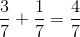Remember, when we are adding fractions we must have common denominators and we only add the numerators.

Example Question #6 : Adding Mixed Numbers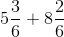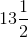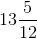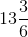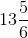Explanation:

When we add mixed numbers, we add whole numbers to whole numbers and fractions to fractions.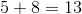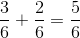Remember, when we are adding fractions we must have common denominators and we only add the numerators.

Example Question #2 : Add And Subtract Mixed Numbers With Like Denominators: Ccss.Math.Content.4.Nf.B.3c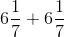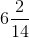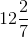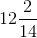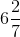Explanation:

When we add mixed numbers, we add whole numbers to whole numbers and fractions to fractions.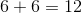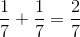Remember, when we are adding fractions we must have common denominators and we only add the numerators.

Example Question #8 : Adding Mixed Numbers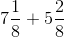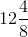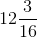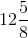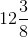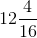Explanation:

When we add mixed numbers, we add whole numbers to whole numbers and fractions to fractions.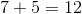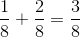Remember, when we are adding fractions we must have common denominators and we only add the numerators.

Example Question #9 : Adding Mixed Numbers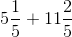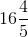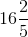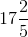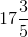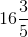Explanation:

When we add mixed numbers, we add whole numbers to whole numbers and fractions to fractions.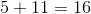Remember, when we are adding fractions we must have common denominators and we only add the numerators.

Example Question #1 : Add And Subtract Mixed Numbers With Like Denominators: Ccss.Math.Content.4.Nf.B.3c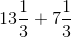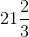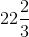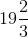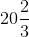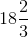Explanation:

When we add mixed numbers, we add whole numbers to whole numbers and fractions to fractions.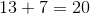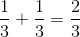Remember, when we are adding fractions we must have common denominators and we only add the numerators.

Example Question #151 : How To Add Fractions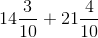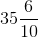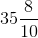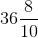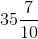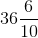Explanation:

When we add mixed numbers, we add whole numbers to whole numbers and fractions to fractions.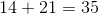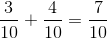Remember, when we are adding fractions we must have common denominators and we only add the numerators.

Example Question #202 : Build Fractions From Unit Fractions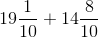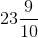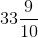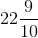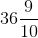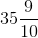Explanation:

When we add mixed numbers, we add whole numbers to whole numbers and fractions to fractions.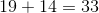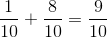Remember, when we are adding fractions we must have common denominators and we only add the numerators.